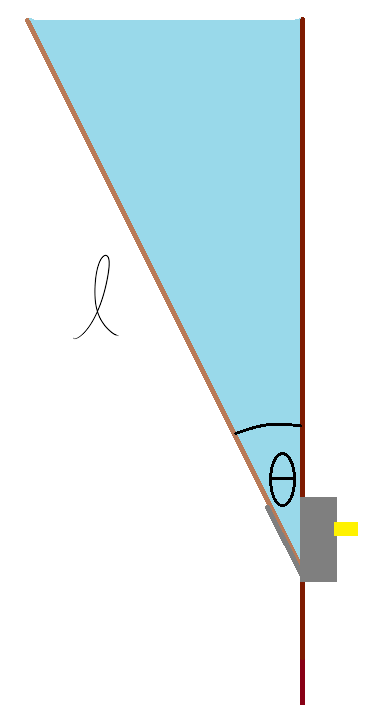# But There Would Be No Mass!In a two-dimensional setting, a water-filled tub of length $\ell$ can be formed by attaching a platform of negligible mass and width to a wall. Using a lever, one can change the angle of elevation, $\theta$, between this platform and the wall.

If the water always levels out at the very top of the tub, find the limit of the tub's center of mass as the angle of elevation increases to approach $90^\circ$. Write your answer as the horizontal distance from the platform's pivot to the center of mass in terms of $\ell$.

Assume that the water is of uniform density.

×